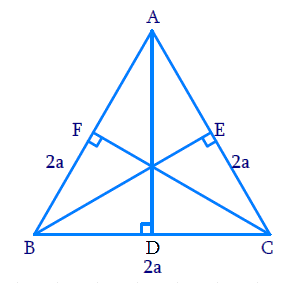In the verge of coronavirus pandemic, we are providing FREE access to our entire Online Curriculum to ensure Learning Doesn't STOP!

# Ex.6.5 Q6 Triangles Solution - NCERT Maths Class 10

Go back to  'Ex.6.5'

## Question

$$ABC$$ is an equilateral triangle of side $$2$$$$a.$$ Find each of its altitudes.

DiagramVideo Solution
Triangles
Ex 6.5 | Question 6

## Text Solution

Reasoning:

We know that in an equilateral triangle perpendicular drawn from vertex to the opposite side, bisects the side.

As we know that, in a right triangle, the square of the hypotenuse is equal to the sum of the squares of the other two sides.

Steps:

In $$\Delta ABC$$

$$A B=B C=C A=2 a$$

$$A D \perp B C$$

\begin{align}\Rightarrow\; B D&=C D=\frac{{1}}{2}BC={a}\end{align}

In $$\Delta ADB$$ ,

\begin{align} A B^{2} &=A D^{2}+B D^{2} \\ A D^{2} &=A B^{2}-B D^{2} \\ &=(2 a)^{2}-a^{2} \\ &=4 a^{2}-a^{2} \\ &=3 a^{2} \\AD &=\sqrt{3} a\\ \Rightarrow A D &=\sqrt{3} a \text { Units } \end{align}

Similarly, we can prove that

$BE=CF=\sqrt{3} a \text { Units }$

Learn from the best math teachers and top your exams

• Live one on one classroom and doubt clearing
• Practice worksheets in and after class for conceptual clarity
• Personalized curriculum to keep up with school